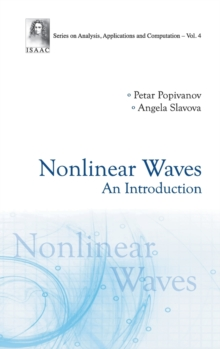Supporting your high street Find out how »
• My AccountNonlinear Waves: An Introduction Hardback

Part of the Series On Analysis, Applications And Computation series

Description

This book deals with equations of mathematical physics as the different modifications of the KdV equation, the Camassa-Holm type equations, several modifications of Burger's equation, the Hunter-Saxton equation and others.

The equations originate from physics but are proposed here for their investigation via purely mathematical methods in the frames of university courses.

More precisely, the authors propose classification theorems for the traveling wave solutions for a sufficiently large class of third order nonlinear PDE when the corresponding profiles develop different kind of singularities (cusps, peaks).

The orbital stability of the periodic solutions of traveling type for mKdV equations are also studied.

Of great interest too is the interaction of peakon type solutions of the Camassa-Holm equation and the solvability of the classical and generalized Cauchy problem for the Hunter-Saxton equation.

The Riemann problem for special systems of conservation laws and the corresponding d-shocks are also considered.

At the end of the book the authors study the interaction of two piecewise smooth waves in the case of two space variables and they verify the appearance of logarithmic singularities.

As it concerns numerical methods in the case of periodic waves the authors apply Cellular Neural Network (CNN) approach.

Information

• Format: Hardback
• Pages: 180 pages
• Publisher: World Scientific Publishing Co Pte Ltd
• Publication Date:
• Category: Mathematical physics
• ISBN: 9789814322126

£60.00

£49.05

on all orders

Pick up orders

from local bookshops